MathScore EduFighter is one of the best math games on the Internet today. You can start playing for free!

## Analyze and Compare Shapes - Sample Math Practice Problems

The math problems below can be generated by MathScore.com, a math practice program for schools and individual families. References to complexity and mode refer to the overall difficulty of the problems as they appear in the main program. In the main program, all problems are automatically graded and the difficulty adapts dynamically based on performance. Answers to these sample questions appear at the bottom of the page. This page does not grade your responses.

See some of our other supported math practice problems.

### Complexity=1, Mode=analy

Answer the questions by selecting any answers that go with the picture.

1.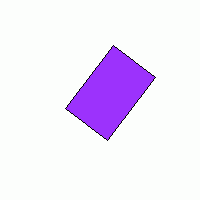Number of sides and corners:
 3
 4
 6
 None of the above
Has sides and corners that are all the same:
 yes
 no
2.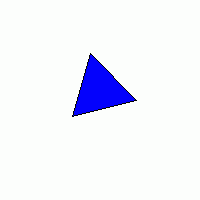Number of sides and corners:
 3
 4
 6
 None of the above
Has sides and corners that are all the same:
 yes
 no

### Complexity=1, Mode=compare

Answer the questions by selecting any answers that go with the picture.

1.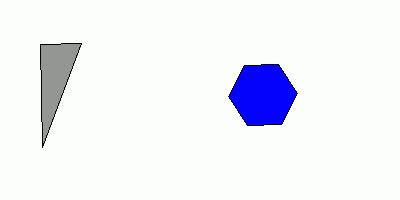What is different about these shapes?
 The number of sides and corners
 One's sides are all the same length; the other doesn't
 None of the above
2.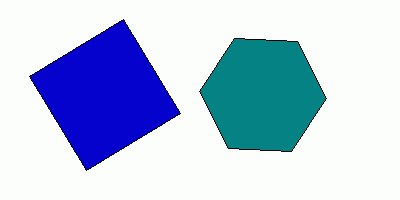How are these shapes alike?
 The number of sides and corners
 Each shape's sides are all the same length
 None of the above

### Complexity=2, Mode=analy

Answer the questions by selecting any answers that go with the picture.

1.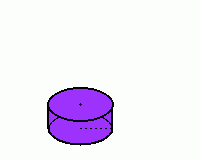Number of sharp corner points:
 1
 2
 6
 8
 12
 None of the above
Number of straight sharp sides/edges:
 1
 2
 6
 8
 12
 None of the above
Number of flat faces:
 1
 2
 6
 8
 12
 None of the above
Has a curved face:
 yes
 no
2.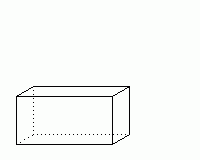Number of sharp corner points:
 1
 2
 6
 8
 12
 None of the above
Number of straight sharp sides/edges:
 1
 2
 6
 8
 12
 None of the above
Number of flat faces:
 1
 2
 6
 8
 12
 None of the above
Has a curved face:
 yes
 no

### Complexity=2, Mode=compare

Answer the questions by selecting any answers that go with the picture.

1.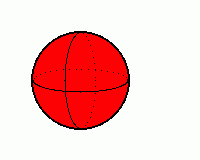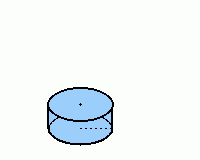How are these shapes alike?
 The number of sides, corners, and faces
 Each has at least one sharp corner point (vertex)
 Each has at least one circular face
 Each has at least one curved surface
 None of the above
2.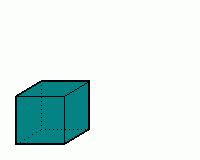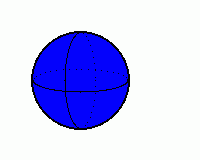What is different about these shapes?
 The number of sides, corners, and faces
 One has a sharp corner point (vertex); the other doesn't
 One has a circular face; the other doesn't
 One has a curved surface; the other doesn't
 None of the above

### Complexity=3, Mode=compare

Answer the questions by selecting any answers that go with the picture.

1.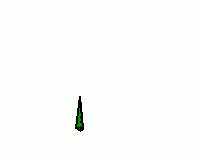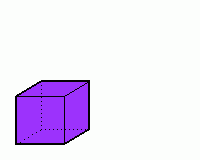How are these shapes alike?
 The number of sides, corners, and faces
 Each has at least one sharp corner point (vertex)
 Each has at least one circular face
 Each has at least one curved surface
 None of the above
2.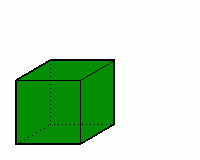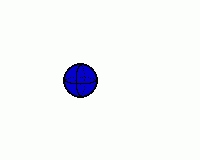How are these shapes alike?
 The number of sides, corners, and faces
 Each has at least one sharp corner point (vertex)
 Each has at least one circular face
 Each has at least one curved surface
 None of the above

### Complexity=1, Mode=analy

Answer the questions by selecting any answers that go with the picture.

1Number of sides and corners:
 3
 4
 6
 None of the above
Has sides and corners that are all the same:
 yes
 no
2Number of sides and corners:
 3
 4
 6
 None of the above
Has sides and corners that are all the same:
 yes
 no

### Complexity=1, Mode=compare

Answer the questions by selecting any answers that go with the picture.

1What is different about these shapes?
 The number of sides and corners
 One's sides are all the same length; the other doesn't
 None of the above
Solution
One shape has 3 sides, and one has 6. The hexagon has equal sides, but the triangle doesn't.
2How are these shapes alike?
 The number of sides and corners
 Each shape's sides are all the same length
 None of the above
Solution
One shape has 4 sides, and one has 6; but each has equal sides.

### Complexity=2, Mode=analy

Answer the questions by selecting any answers that go with the picture.

1Number of sharp corner points:
 1
 2
 6
 8
 12
 None of the above
Number of straight sharp sides/edges:
 1
 2
 6
 8
 12
 None of the above
Number of flat faces:
 1
 2
 6
 8
 12
 None of the above
Has a curved face:
 yes
 no
Solution
If it looks like there are straight edges, notice they are not sharp. You can roll a piece of paper into a cylinder, which needs no folding, and folding is what creates sharp sides.
(You can still call it a cylinder without the circular bases.)
2Number of sharp corner points:
 1
 2
 6
 8
 12
 None of the above
Number of straight sharp sides/edges:
 1
 2
 6
 8
 12
 None of the above
Number of flat faces:
 1
 2
 6
 8
 12
 None of the above
Has a curved face:
 yes
 no
Solution
For faces, there are front, back, left, right, top, bottom -- that makes 6.
For sides, there are 4 making up the top face, 4 making up the bottom (8 corners), and 4 that are connecting pairs of top-bottom corners to make up the sides -- that makes 12.

### Complexity=2, Mode=compare

Answer the questions by selecting any answers that go with the picture.

1How are these shapes alike?
 The number of sides, corners, and faces
 Each has at least one sharp corner point (vertex)
 Each has at least one circular face
 Each has at least one curved surface
 None of the above
Solution
A cylinder, like a sphere, has no sharp corners. it has 2 circular faces, but a sphere has none (no flat faces).
2What is different about these shapes?
 The number of sides, corners, and faces
 One has a sharp corner point (vertex); the other doesn't
 One has a circular face; the other doesn't
 One has a curved surface; the other doesn't
 None of the above
Solution
A sphere is very different from a cube. It has no sharp corners or sides, or (flat) faces. It is just 1 curved surface, which a cube does not have.

### Complexity=3, Mode=compare

Answer the questions by selecting any answers that go with the picture.

1How are these shapes alike?
 The number of sides, corners, and faces
 Each has at least one sharp corner point (vertex)
 Each has at least one circular face
 Each has at least one curved surface
 None of the above
Solution
The cone has 1 corner point and 2 surfaces: a circular face and a curved surface.
2How are these shapes alike?
 The number of sides, corners, and faces
 Each has at least one sharp corner point (vertex)
 Each has at least one circular face
 Each has at least one curved surface
 None of the above
Solution
A sphere is very different from a cube. It has no sharp corners or sides, or (flat) faces. It is just 1 curved surface, which a cube does not have.